## Berge Tangle

Here’s a tangle in S^2 x I.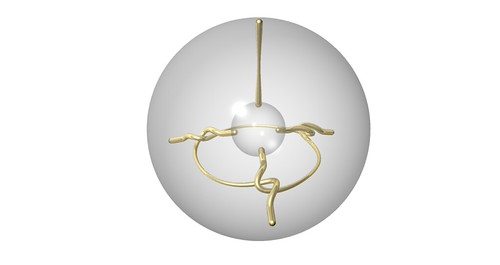One may show that it’s not equivalent to the trivial product tangle.

Here’s another view that shows off a 3-fold symmetry.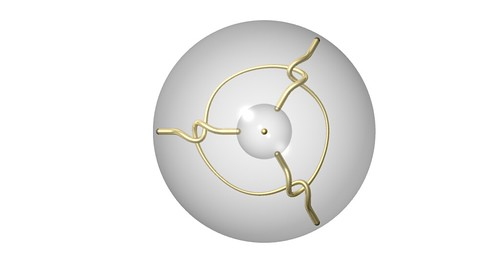We’re gonna fill the “inner” sphere with a trivial tangle in three different ways.

These are each trivial (2-strand tangles in the ball) because the arcs can be pushed onto the boundary, fixing their endpoints.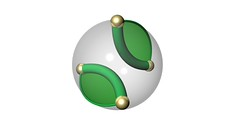With the fixed endpoints, they are what we call rational tangles. And we can see that the three are “mutually distance 1”.

These filling tangles also respect that 3-fold symmetry.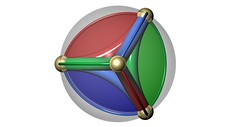So here’s the three fillings…And of course these three filled tangles are related by that 3-fold symmetry.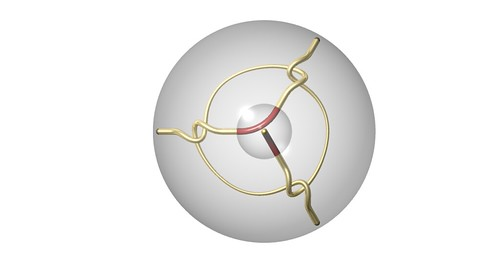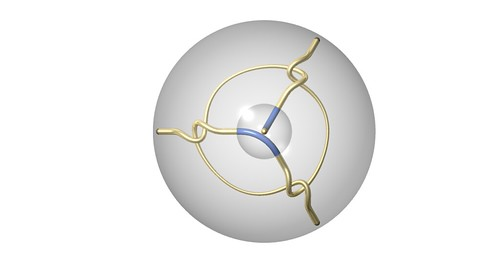The curious thing about this triple of fillings is that each filling produces a rational tangle. This is perhaps easier to see by letting the endpoints of one of the resulting 2-strand tangles in a ball wander around as you untwist its strands until you end up with something that is clearly a trivial tangle.

Because each of these are rational tangles, that means their arcs can be pushed onto the boundary sphere, keeping the endpoints fixed. Here’s a couple of views of the result of this for the red filling. (Check the Flickr set for the others and more pics of these tangles.)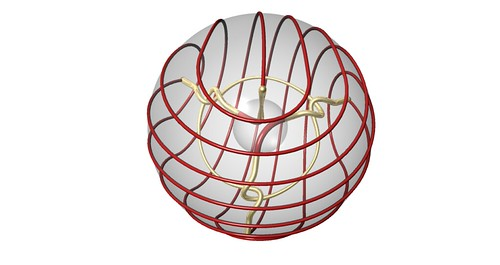Forgetting about the inside, here are the two arcs isotoped to the boundary.Now looking at each arc individually.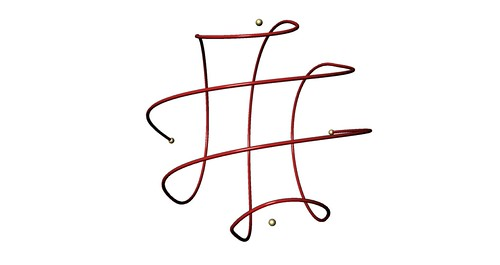Here’s the red and green together.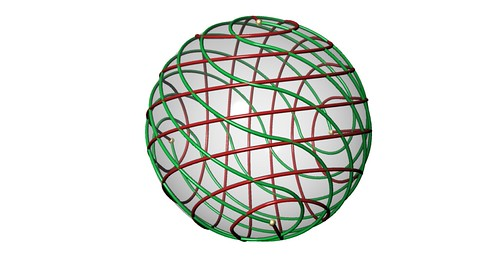Here’s one red arc with one green arc.And here’s the red, green, and blue together. (What a mess!)So as one may have guessed from the title of this post, the branched double cover of the initial tangle in S^2 x I is the so-called Berge Manifold. It’s the unique hyperbolic manifold for which there are three mutually distance 1 fillings of a cusp that produce a solid torus. And as one may recall, the branched double cover of a rational tangle is a solid torus.

~ by Ken Baker on July 17, 2010.

### 3 Responses to “Berge Tangle”

1. Nice pictures! If you erase the trivial arc which is invariant under the 3-fold symmetry, you get Thurston’s tripus manifold. The tripus has a decomposition into two truncated tetrahedra, with this arc as the only edge. I suppose this is one way to show that the tangle is non-trivial. Berge’s manifold also has a nice regular ideal tetrahedron decomposition.